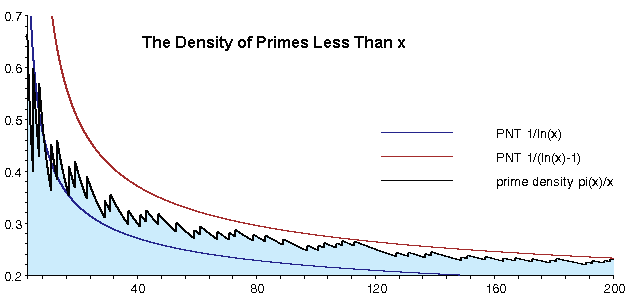# Digital Sum part 2The digital root of a number is obtained by adding together the digits of that number, and repeating that process until a number is arrived at that is less than $10$.

For example,for $29953$ the digital root is $29953 \rightarrow 28\rightarrow 10\rightarrow 1$.

Consider the set $P$ of all prime numbers less than two million.If $x$ is the percentage of numbers in $P$ with a digital root of 2,what is the value of $\left\lfloor 1000x \right\rfloor$ ?

Details and assumptions

$\left\lfloor x \right\rfloor$ is the floor function and it returns the greatest integer less or equal to $x$. ie $\left\lfloor 1.234 \right\rfloor =1$

×

Problem Loading...

Note Loading...

Set Loading...